Next: Exercises Up: Radiation and Scattering Previous: Thomson Scattering

# Rayleigh Scattering

Let us now consider the scattering of electromagnetic radiation by a harmonically bound electron: for instance, an electron orbiting an atomic nucleus. We have seen in Section 7.3 that such an electron satisfies an equation of motion of the form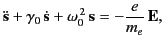(1289)

where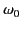is the characteristic oscillation frequency of the electron, and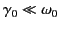is the damping rate of such oscillations. Assuming an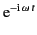time dependence of both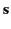and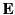, we find that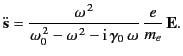(1290)

It follows, by analogy with the analysis in the previous section, that the total scattering cross-section is given by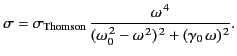(1291)

The angular distribution of the radiation is the same as that in the case of a free electron.

The maximum value of the cross-section (1293) is obtained when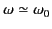: that is, for resonant scattering. In this case, the scattering cross-section can become very large. In fact,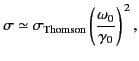(1292)

which is generally far greater than the Thomson scattering cross-section.

For the case of strong binding,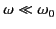, and Equation (1293) reduces to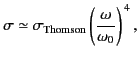(1293)

giving a scattering cross-section that depends on the inverse fourth power of the wavelength of the incident radiation. The cross-section (1295) is known as the Rayleigh scattering cross-section, and is appropriate to the scattering of visible radiation by gas molecules. This is the basis of Rayleigh's famous explanation of the blue sky. The air molecules of the atmosphere preferentially scatter the shorter wavelength blue components out of white'' sunlight which grazes the atmosphere. Conversely, sunlight viewed directly through the long atmospheric path at sunset appears reddened. The Rayleigh scattering cross-section is much less than the Thompson scattering cross-section (for). However, this effect is offset to some extent by the fact that the density of neutral molecules in a gas (e.g., the atmosphere) is much larger than the density of free electrons typically encountered in a plasma.Next: Exercises Up: Radiation and Scattering Previous: Thomson Scattering
Richard Fitzpatrick 2014-06-27